# The REG Procedure

### ODS Graphics

Subsections:

Statistical procedures use ODS Graphics to create graphs as part of their output. ODS Graphics is described in detail in Chapter 21: Statistical Graphics Using ODS.

Before you create graphs, ODS Graphics must be enabled (for example, by specifying the ODS GRAPHICS ON statement). For more information about enabling and disabling ODS Graphics, see the section Enabling and Disabling ODS Graphics in Chapter 21: Statistical Graphics Using ODS.

The overall appearance of graphs is controlled by ODS styles. Styles and other aspects of using ODS Graphics are discussed in the section A Primer on ODS Statistical Graphics in Chapter 21: Statistical Graphics Using ODS.

The following sections describe the ODS graphical displays produced by PROC REG.

#### Diagnostics Panel

The "Diagnostics Panel" provides a display that you can use to get an overall assessment of your model. See Figure 97.9 for an example.

The panel contains the following plots:

• residuals versus the predicted values

• externally studentized residuals (RSTUDENT) versus the predicted values

• externally studentized residuals versus the leverage

• normal quantile-quantile plot (Q-Q plot) of the residuals

• dependent variable values versus the predicted values

• Cook’s D versus observation number

• histogram of the residuals

• "Residual-Fit" (or RF) plot consisting of side-by-side quantile plots of the centered fit and the residuals

• box plot of the residuals if you specify the STATS=NONE suboption

Patterns in the plots of residuals or studentized residuals versus the predicted values, or spread of the residuals being greater than the spread of the centered fit in the RF plot, are indications of an inadequate model. Patterns in the spread about the 45-degree reference line in the plot of the dependent variable values versus the predicted values are also indications of an inadequate model.

The Q-Q plot, residual histogram, and box plot of the residuals are useful for diagnosing violations of the normality and homoscedasticity assumptions. If the data in a Q-Q plot come from a normal distribution, the points will cluster tightly around the reference line. A normal density is overlaid on the residual histogram to help in detecting departures form normality.

Following Rawlings, Pantula, and Dickey (1998), reference lines are shown on the relevant plots to identify observations deemed outliers or influential. Observations whose externally studentized residual magnitudes exceed 2 are deemed outliers. Observations whose leverage value exceeds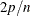or whose Cook’s D value exceeds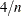are deemed influential (p is the number of regressors including the intercept, and n is the number of observations used in the analysis). If you specify the LABEL suboption of the PLOTS=DIAGNOSTICS option, then the points deemed outliers or influential are labeled on the appropriate plots.

Fit statistics are shown in the lower right of the plot and can be customized or suppressed by using the STATS= suboption of the PLOTS=DIAGNOSTICS option.

#### Residuals by Regressor Plots

Panels of plots of the residuals versus each of the regressors in the model are produced by default. Patterns in these plots are indications of an inadequate model. To help in detecting patterns, you can use the SMOOTH= suboption of the PLOTS=RESIDUALS option to add loess fits to these residual plots. See Output 97.1.7 for an example.

#### Fit and Prediction Plots

A fit plot consisting of a scatter plot of the data overlaid with the regression line, as well as confidence and prediction limits, is produced for models depending on a single regressor. Fit statistics are shown to the right of the plot and can be customized or suppressed by using the STATS= suboption of the PLOTS=FIT option.

When a model contains more than one regressor, a fit plot is not appropriate. However, if all the regressors in the model are transformations of a single variable in the input data set, then you can request a scatter plot of the dependent variable overlaid with a fit line and confidence and prediction limits versus this variable. You can also plot residuals versus this variable. You request these plots, shown in a panel, with the PLOTS=PREDICTION option. See Figure 97.14 for an example.

#### Influence Plots

In addition to the "Cook’s D Plot" and the "RStudent By Leverage Plot," you can request plots of the DFBETAS and DFFITS statistics versus observation number by using the PLOTS=DFBETAS and PLOTS=DFFITS options. You can also obtain partial regression leverage plots by using the PLOTS=PARTIAL option. See the section Influence Statistics for examples of these plots and details about their interpretation.

#### Ridge and VIF Plots

When you use ridge regression, you can request plots of the variance inflation factor (VIF) values and standardized ridge estimates by ridge values for each coefficient with the PLOTS=RIDGE option. See Example 97.5 for examples.

#### Variable Selection Plots

When you request variable selection by using the SELECTION= option in the MODEL statement, you can request plots of fit criteria for the models examined by using the PLOTS=CRITERIA option. The fit criteria are displayed versus the step number for the FORWARD, BACKWARD, and STEPWISE selection methods and the step at which the optimal value of each criterion is obtained is indicated using a "Star" marker. For the all-subset-based selection methods (SELECTION=RSQUARE|ADJRSQ|CP), the fit criteria are displayed versus the number of observations in the model.

The criteria are shown in a panel, but you can use the UNPACK suboption of the PLOTS=CRITERIA option to obtain separate plots for each criterion. You can also use the LABEL suboption of the PLOTS=CRITERIA option to request that optimal models be labeled on the plots. Example 97.2 provides several examples.

#### Heat Maps

PROC REG can produce either fit and residual scatter plots for smaller data sets or heat maps for larger data sets. The global plot option MAXPOINTS= max heat-max controls which of these are produced. When the number of points exceeds the value of max but does not exceed the value of heat-max divided by the number of independent variables, heat maps are displayed instead of scatter plots for the fit and residual plots. All other graphs are suppressed when the number of points exceeds max. The default is MAXPOINTS=5000 150000. These cutoffs are ignored if you specify MAXPOINTS=NONE. The following statements create both scatter plots and heat maps with artificial data:

```data x;
do i = 1 to 25000;
x = 2 * normal(104);
y = x + sin(x * 2) + 3 * normal(104);
output;
end;
run;

ods graphics on;

proc reg data=x plots(maxpoints=30000);
model y = x;
run; quit;

proc reg data=x;
model y = x;
run; quit;
```

Scatter plots are displayed in Figure 97.54, and heat maps are displayed in Figure 97.55.

Figure 97.54: Scatter Plot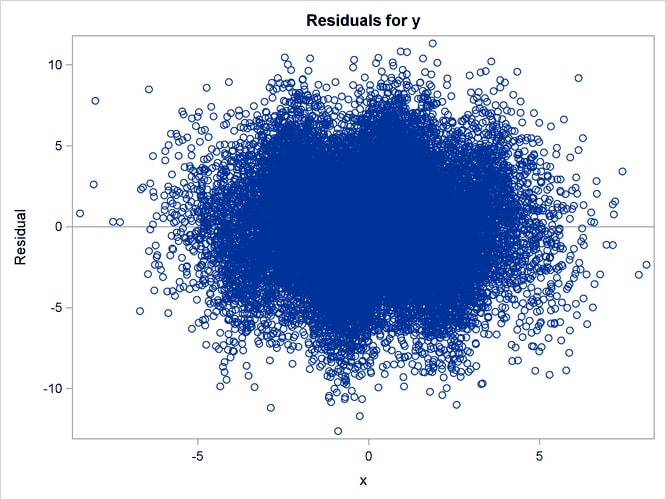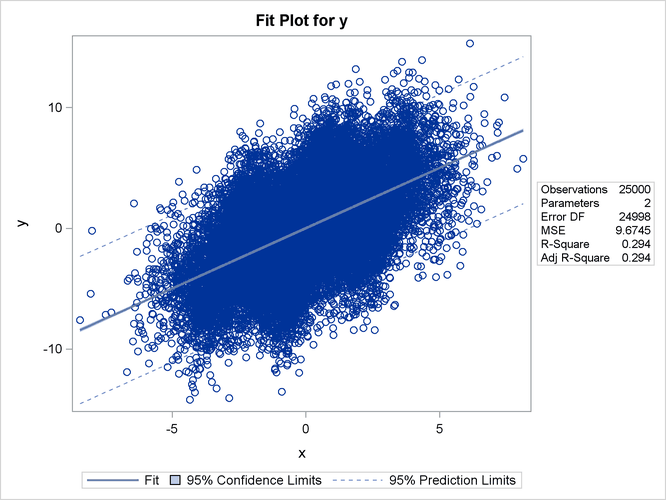Figure 97.55: Heat Map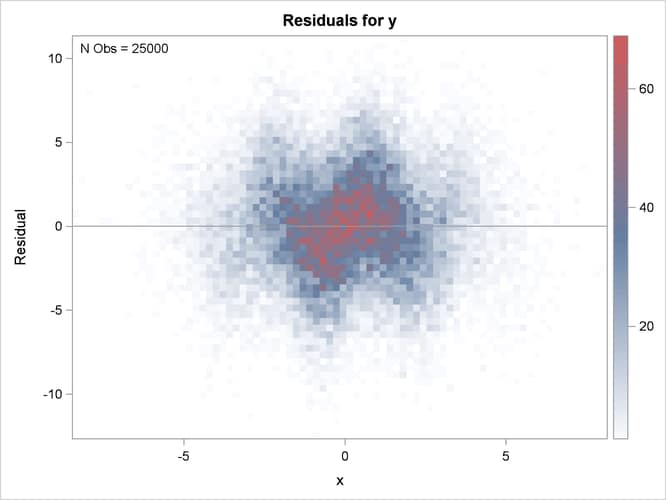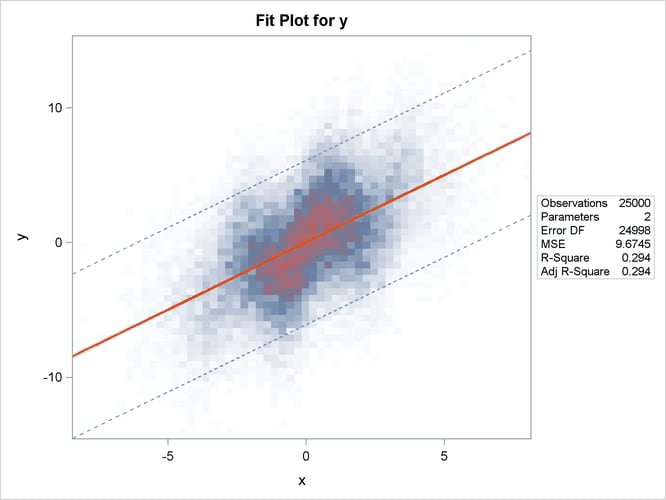The heat maps show more clearly that the sine function is not fit well by the linear fit function.

#### ODS Graph Names

PROC REG assigns a name to each graph it creates using ODS. You can use these names to reference the graphs when using ODS. The names are listed in Table 97.13.

Table 97.13: ODS Graphical Displays Produced by PROC REG

ODS Graph Name

Plot Description

PLOTS Option

Adjusted R-square statistic for models examined doing variable selection

AICPlot

AIC statistic for models examined doing variable selection

AIC

BICPlot

BIC statistic for models examined doing variable selection

BIC

CooksDChart

Cook’s D chart

PLOTS=RESIDUALCHART(UNPACK)

CooksDPlot

Cook’s D statistic versus observation number

COOKSD

CPPlot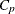statistic for models examined doing variable selection

CP

DFFITSPlot

DFFITS statistics versus observation number

DFFITS

DFBETASPanel

Panel of DFBETAS statistics versus observation number

DFBETAS

DFBETASPlot

DFBETAS statistics versus observation number

DFBETAS(UNPACK)

DiagnosticsPanel

Panel of fit diagnostics

DIAGNOSTICS

FitPlot

Regression line, confidence limits, and prediction limits overlaid on a scatter plot of the data

FIT, MAXPOINTS= max not exceeded

FitPlot

Regression line overlaid on a heat map of the data

FIT, MAXPOINTS= max exceeded

ObservedByPredicted

Dependent variable versus predicted values

OBSERVEDBYPREDICTED

PartialPlot

Partial regression plot

PARTIAL

PredictionPanel

Panel of residuals and fit versus specified variable

PREDICTIONS

PredictionPlot

Regression line, confidence limits, and prediction limits versus specified variable

PREDICTIONS(UNPACK)

PredictionResidualPlot

Residuals versus specified variable

PREDICTIONS(UNPACK)

QQPlot

Normal quantile plot of residuals

QQ

ResidualBoxPlot

Box plot of residuals

BOXPLOT

ResidualByPredicted

Residuals versus predicted values

RESIDUALBYPREDICTED

ResidualHistogram

Histogram of fit residuals

RESIDUALHISTOGRAM

ResidualPlot

Scatter plot of residuals versus regressor

RESIDUALS, MAXPOINTS= max not exceeded

ResidualPlot

Heat map of residuals versus regressor

RESIDUALS, MAXPOINTS= max exceeded

RFPlot

Side-by-side plots of quantiles of centered fit and residuals

RF

RidgePanel

Plot of VIF and ridge traces

RIDGE

RidgePlot

Plot of ridge traces

RIDGE(UNPACK)

RSquarePlot

R-square statistic for models examined doing variable selection

RSQUARE

RStudentByLeverage

Studentized residuals versus leverage

RSTUDENTBYLEVERAGE

RStudentByPredicted

Studentized residuals versus predicted values

RSTUDENTBYPREDICTED

SBCPlot

SBC statistic for models examined doing variable selection

SBC

SelectionCriterionPanel

Panel of fit statistics for models examined doing variable selection

CRITERIA

StudResCooksDChart

Studentized residual and Cook’s D chart

PLOTS=RESIDUALCHART

StudentResChart

Studentized residual chart

PLOTS=RESIDUALCHART(UNPACK)

VIFPlot

Plot of VIF traces

RIDGE(UNPACK)